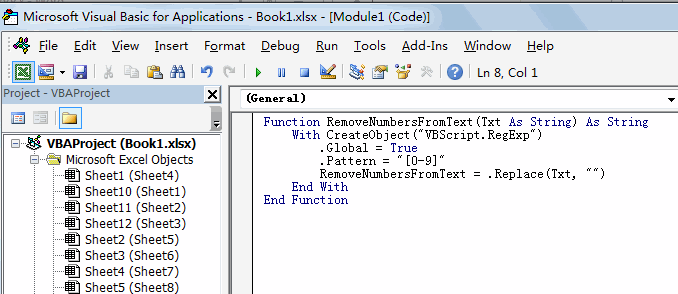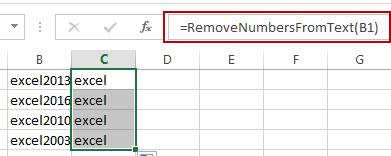# How to Remove Numbers from Text in Excel

This post will guide you how to remove all numbers from a text string in one cell in Excel. How do I strip all numeric characters from a given cell with a formula in Excel. How do I remove numbers from cells with text using a User Defined Function in Excel.

## Remove Numbers from Text Using Formula

Assuming that you have a list of data in range B1:B4 which contain test string values. And you want to look for a formula to remove all numbers from text string in given range of cells. You can use an array formula based on the TEXTJOIN function. Like this:

`=TEXTJOIN("",TRUE,IF(ISERR(MID(B1,ROW(INDIRECT("1:100")),1)+0),MID(B1,ROW(INDIRECT("1:100")),1),""))`

Type this formula into a blank cell and press Ctrl + Shift + Enter keys to change the formula as array formula.

## Remove Numbers from Text Using User Defined Function

You can also write a User Defined Function with VBA code to achieve the same result of removing all numbers from text string in the selected range of cells in Excel. Here are the steps:

#1 open your excel workbook and then click on “Visual Basic” command under DEVELOPER Tab, or just press “ALT+F11” shortcut.#2 then the “Visual Basic Editor” window will appear.

#3 click “Insert” ->”Module” to create a new module.#4 paste the below VBA code into the code window. Then clicking “Save” button.#5 back to the current worksheet, then type the following formula in a blank cell, and then press Enter key.

=RemoveNumbersFromText(B1)

#6 drag the AutoFill handle over other cells to  remove numbers from text in one cell.### Related Functions

• Excel ROW function
The Excel ROW function returns the row number of a cell reference.The ROW function is a build-in function in Microsoft Excel and it is categorized as a Lookup and Reference Function.The syntax of the ROW function is as below:= ROW ([reference])….
• Excel IF function
The Excel IF function perform a logical test to return one value if the condition is TRUE and return another value if the condition is FALSE. The IF function is a build-in function in Microsoft Excel and it is categorized as a Logical Function.The syntax of the IF function is as below:= IF (condition, [true_value], [false_value])….
• Excel MID function
The Excel MID function returns a substring from a text string at the position that you specify.The syntax of the MID function is as below:= MID (text, start_num, num_chars)…
• Excel INDIRECT function
The Excel INDIRECT function returns the cell reference based on a text string, such as: type the text string “A2” in B1 cell, it just a text string, so you can use INDIRECT function to convert text string as cell reference….
Related Posts

How to Extract First Letter from Each Word in a Cell in Excel

This post will guide you how to extract first letter from each word in a given cell in Excel. How do I extract the first letter of each word in a range of names in your current worksheet in Microsoft ...

How to ignore Blank Cells while Concatenating cells in Excel

This post will guide you how to concatenate cells but ignore all blank cells in your worksheet in Excel. How do I concatenate cells but ignore blank cells with a formula in Excel. How to create a concatenate formula to ...

How to Calculate the Average Excluding the Smallest & Highest Numbers in Excel

Calculating the average for a batch of data is frequently used in our daily life. But for some cases like statistic the average score in a competition, or price analysis, we often calculate the average excluding the smallest and highest ...

How to Get the Maximum or Minimum Absolute Value in Excel

It is easy to find the maximum or minimum value in a batch of data in excel, but if this batch of numbers contains both positive and negative numbers, the maximum or minimum absolute value cannot be found out by ...

How to Count Only Numbers in Bold in a Range of Cells in Excel

This post will guide you how to Count only numbers with bold style in a range of cells in Excel. How do I Count on cells with bold font within a range of cells using User defined function in Excel ...

How to Sum Only Numbers in Bold in a Range of Cells in Excel

This post will guide you how to sum only numbers with bold style in a range of cells in Excel. How do I sum on cells with bold font within a range of cells using User defined function in Excel ...

How to Filter Formula in Excel

This post will guide you how to filter your data with an Formula in Excel. How do I filter cells with formulas through a User defined function in Excel 2013/2016. Filter Formula Assuming that you have a list of data ...

How to Count Cells by Font Color in Excel

This post will guide you how to count cells by font color in Excel. How do I Count the number of cells with specific cell Font color by using a User Defined function in Excel. Count Cells by Font Color ...

How to Highlight Cells Containing Formulas in Excel

This post will guide you how to highlight cells in which contain formulas using Conditional Formatting feature in Excel. How do I conditionally format a cell if it contains formula using a User defined function in combination with Conditional Formatting ...

How to Extract Number from Text String in Excel

This post will guide you how to extract number from a given test string in Excel. How do I extract all numbers from string using a formula in Excel. How to get all number from a given test string using ...

Sidebar